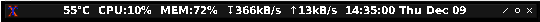# Command to read CPU, GPU temperature?

Any idea which command should we use to get current temp readings on GPU and CPU?

Thanks

Hi,
cat /sys/class/thermal/thermal_zone0/temp
cat /sys/class/thermal/thermal_zone1/temp
to get temps from the 2 thermal zones of the soc (they appear to be related)
I don’t know if it’s possible to get either CPU or GPU temp.

You could also try:
cat /sys/devices/system/cpu/cpu0/cpufreq/cpuinfo_cur_freq

cat /sys/devices/system/cpu/cpu5/cpufreq/cpuinfo_cur_freq
to get each core frequency and understand if the core is throttling / overheating.

2 Likes

you should note that even though the frequency is dynamic it is kinda of fixed to a few specific freqencies,especially the 100MHz multiples, so you will see frequencies like 2218 MHz, 2118MHz, 2018 Mhz, 1918 MHz etc.

The system shows that the CPU is always running at full speed. 1800MHz on the A53 cores and 2200MHz on the A72 Cores. Is there trick to get the real values?

Well if your device is not throttling it will be in the highest clock rate, this is because the governor is set to Performance by default, you can set it to be in the powersave mode and see that that your clock rate suddenly drops when Idle…

Thanks, thats true the governor is set to “performance”.
How canI set it to “interactive”? I use Ubunutu 18.04 and cpuwoer does not work with provided kernel.

1 Like

I think you can try this, it is a permanent fix:

``````sudo sed -i 's/^GOVERNOR=.*/GOVERNOR="interactive"/' /etc/init.d/cpufrequtils
``````
3 Likes

hi, yes, most likely it is enough to change the system settings for the processor “interactive”

1 Like

Is interactive running stable?

interactive is usually the default for Android and is considered popular.

Interactive - “productive” kernel mode. Modified kernel mode ondemand, which changes the core frequency faster, but unlike ondemand, it is allowed to spend more time at the maximum frequency. Therefore less energy efficient.

Assuming the units returned are in thousandths of a degree C?

I wrote the following script to display temp, cpu, memory use, download speed, upload speed and time/date in the terminal window title. This saves me the screen space that a system monitor like gkrellm takes-up.

Modify to suit your taste. and enjoy``````#!/bin/bash

#interface to monitor
myif="eth0"

mydelay=\${1:-3}         # Default delay 3 sec, or pass a number for update time

function getcpustat() {
sed -ne '/^cpu  */s/^cpu  *//p' /proc/stat
# that is:
# -n to not print lines by default
# for lines matching /^cpu  */, delete /^cpu  */, and print the line
}

function extract() {
# example 1st param: "437797 1219 185341 549955584 58662 4 7083 0 0 0"
# example 2nd param: 1, 2, 3, ... (the n-th column to use)
# maybe can use readarray to make what extract() does simpler
n=\$2          # saving the position param
set -- \$1     # reset positional params from \$1, so that \$1=437797, \$2=1219, ...
echo \${!n}    # echo the n-th positional param
}

function change() {
local e=\$(extract "\$endstat" \$1)
local b=\$(extract "\$startstat" \$1)
echo \$(( \$e - \$b ))
}

function mempct() {
echo \$(free | awk '/Mem/{printf("%2d"), \$3/\$2*100}')
}

#Setup the begin rate statistics
startstat=\$(getcpustat)
idowna=\$(( \$(< /sys/class/net/eth0/statistics/rx_bytes) / 1024 ))
iupa=\$(( \$(< /sys/class/net/eth0/statistics/tx_bytes) / 1024 ))
sleep "\$mydelay"

GREN="\033[0;32m"
#now loop and update our stats
while :
do
#End rate statistics
endstat=\$(getcpustat)           # get end cpu stats
idownb=\$(( \$(< /sys/class/net/eth0/statistics/rx_bytes) / 1024 ))
iupb=\$(( \$(< /sys/class/net/eth0/statistics/tx_bytes) / 1024 ))
(( iratedown = (idownb - idowna)/mydelay ))
(( irateup = (iupb - iupa)/mydelay ))

# Calculate cpu percent total PCT.
myusr=\$(change 1)
mysys=\$(change 3)
myidle=\$(change 4)
myiow=\$(change 5)
myact=\$(( myusr + mysys + myiow ))
mytot=\$((myact + myidle))
mypct=\$(( myact * 100 / mytot ))

# Get CPU temperature, divide appropriately.
mytemp=\$(( \$(< /sys/class/thermal/thermal_zone0/temp) / 1000 ))

# Get mem stats
mymem=\$(mempct)

mydate=\$(date +%H:%M:%S\ %a\ %b\ %d | { IFS=: read h m s a b d; printf "\$h:\$m:\$s \$a \$b \$d" ; })

#Print stats. To reduce spam, comment-out a printf and adjust \$mycolu
#printf -v result '%d°C  CPU:%d%% %b MEM:%d%%  ↧%dkB/s  ↑%dkB/s  %s' "\${mytemp}" "\${mypct}" "\${GREN}" "\${mymem}" "\${iratedown}" "\${irateup}" "\${mydate}"
#tobar='\e]0;%s\x07' # Code to print to terminal title bar
#bad - no colorsprintf -v result "%b %d°C  CPU:%d%% %b MEM:%d%%  ↧%dkB/s  ↑%dkB/s  %s" "\${tobar}" "\${mytemp}" "\${mypct}" "\${GREN}" "\${mymem}" "\${iratedown}" "\${irateup}" "\${mydate}"
printf -v result "%d°C  CPU:%d%%  MEM:%d%%  ↧%dkB/s  ↑%dkB/s  %s" "\${mytemp}" "\${mypct}" "\${mymem}" "\${iratedown}" "\${irateup}" "\${mydate}"
printf '\e]0;%s\x07' "\${result}"        # print to term window

#Start rate statistics
STARTSTAT=\$(getcpustat)
idowna=\$(( \$(< /sys/class/net/eth0/statistics/rx_bytes) / 1024 ))
iupa=\$(( \$(< /sys/class/net/eth0/statistics/tx_bytes) / 1024 ))
sleep "\$mydelay"
done

``````

Note the cpu stat isnt including nice’d jobs.

5 Likes